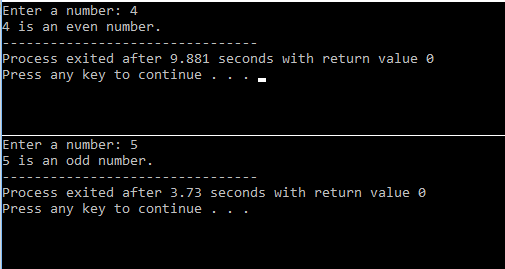# C Program to find whether a number is even or odd.

Hey there!

Welcome to ClearUrDoubt.com.

In this post, we will look at a C program to find whether a given number is even or odd.

Even Number: A number which is divisible by 2
Odd Number: A number which is not divisible by 2

To find whether a number is even or odd, we need to check the remainder when we divide the given number with 2.

This can be achieved using Modulus operator(“%”) in C. This operator gives the remainder of the division expression.

Eg.
10 % 3 = 1
11 % 3 = 2
12 % 3 = 0

Here is the C program:

Output:Happy Learning! 🙂